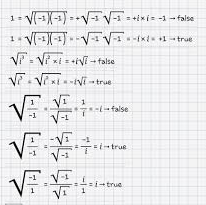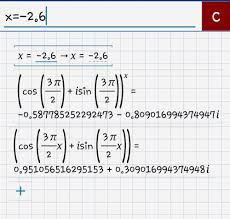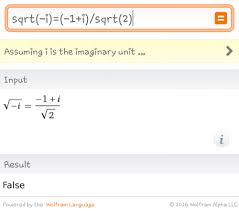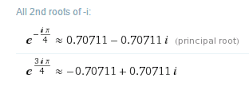IntMath Home » Forum home » Complex Numbers » square roots!

# square roots! [Solved!]

### My question

Hello Murray,
I wanted to ask you a strange question that I found it little weird.. When we first learned about square roots we had never encountered complex numbers, so the only objects that had sqare roots were positive numbers. In this case, sqrt(a/b) = sqrt(a) / sqrt(b) is always true .. But it fails to remain true after we extend the definition of "square root" to allow the square roots of negative and complex numbers.
Where the correct rule was
sqrt(a/b) = + or - sqrt(a) / sqrt(b)
Same thing for
sqrt(a*b) = + or - sqrt(a) * sqrt(b)
If a and b are positive real numbers the equation with a + sign will take its place!
This happened when we tried to calculate the following(attachment):**Notice the minus that must be included somtimes .. so the question is : Are there any logical explanation for when we must have - or + ?? Or it just had to be randomly taken and wish it would be true?

### Relevant page

Sqrt (-1) * sqrt (-1) | Physics Forums - The Fusion of Science and Community

### What I've done so far

(Work included as a photo in the Question!)

X

Hello Murray,
I wanted to ask you a strange question that I found it little weird.. When we first learned about square roots we had never encountered complex numbers, so the only objects that had sqare roots were positive numbers. In this case, sqrt(a/b) = sqrt(a) / sqrt(b) is always true .. But it fails to remain true after we extend the definition of "square root" to allow the square roots of negative and complex numbers.
Where the correct rule was
sqrt(a/b) =  + or -   sqrt(a) / sqrt(b)
Same thing for
sqrt(a*b) =  + or -  sqrt(a) * sqrt(b)
If a and b are positive real numbers the equation with a + sign will take its place!
This happened when we tried to calculate the following(attachment):

<img src="/forum/uploads/imf-4733-screenshot_2016-07-03-03-57-09-1.png" width="206" height="205" alt="complex-numbers" />

**Notice the minus that must be included somtimes .. so the question is : Are there any logical explanation for when we must have - or + ?? Or it just had to be randomly taken and wish it would be true?
Relevant page

<a href="https://www.physicsforums.com/threads/sqrt-1-sqrt-1.571842/">Sqrt (-1) * sqrt (-1) | Physics Forums - The Fusion of Science and Community</a>

What I've done so far

(Work included as a photo in the Question!)

## Re: square roots!

Hello Nour

It's good you are asking such questions - mathematics is not always as consistent as (some) math teachers make it out to be.

Some of these issues also came up in Rika's post, here: Rules for radicals

The main problem is we should always define the conditions for such expressions to be true.

You wrote

sqrt(a/b) = sqrt(a) / sqrt(b) is always true.

Well, only if a>0, b>0.

You then said:

sqrt(a/b) = +- sqrt(a) / sqrt(b)

sqrt(a*b) = +- sqrt(a) * sqrt(b)

Actually, these 2 are not correct. A square root only gives us one positive result.

See this (and the discussion following):

Now to the equations in your image. (You are encouraged to use the math entry system - it makes it easier to discuss.)

For the first 2, neither statement is correct because by convention, we multiply under the square root first. (See Examples 3d and 3e on this page: 1. Basic Definitions of Complex Numbers)

For your 3rd and 4th equations, once again neither is correct because we should multiply under the square root first.

So it should be:

sqrt(i^3) = sqrt(-i)

Now, that provides us with a challenge. We'll need to use DeMoivre's theorem:

-i = cos((3pi)/2) + i sin((3pi)/2)

sqrt(-i) = (cos((3pi)/2) + i sin((3pi)/2))^(1/2)

= cos((3pi)/4) + i sin((3pi)/4)

= (-1+i)/sqrt(2)

Do you think you can sort out your last 3 equations now?

X

Hello Nour

It's good you are asking such questions - mathematics is not always as consistent as (some) math teachers make it out to be.

The main problem is we should always define the conditions for such expressions to be true.

You wrote

sqrt(a/b) = sqrt(a) / sqrt(b) is always true.

Well, only if a&gt;0, b&gt;0.

You then said:

sqrt(a/b) = +- sqrt(a) / sqrt(b)

sqrt(a*b) = +- sqrt(a) * sqrt(b)

Actually, these 2 are not correct. A square root only gives us <b>one</b> positive result.

See this (and the discussion following):

Now to the equations in your image. (You are encouraged to use the <a href="http://www.intmath.com/forum/entering-math-graphs-images-41/how-to-enter-math:91">math entry system</a> - it makes it easier to discuss.)

For the first 2, neither statement is correct because by convention, we multiply under the square root first. (See Examples 3d and 3e on this page: <a href="/complex-numbers/1-basic-definitions.php">1. Basic Definitions of Complex Numbers</a>)

For your 3rd and 4th equations, once again neither is correct because we should multiply under the square root first.

So it should be:

sqrt(i^3) = sqrt(-i)

Now, that provides us with a challenge. We'll need to use <a href="http://www.intmath.com/complex-numbers/7-powers-roots-demoivre.php">DeMoivre's theorem</a>:

-i = cos((3pi)/2) + i sin((3pi)/2)

sqrt(-i) = (cos((3pi)/2) + i sin((3pi)/2))^(1/2)

= cos((3pi)/4) + i sin((3pi)/4)

=  (-1+i)/sqrt(2)

Do you think you can sort out your last 3 equations now?

## Re: square roots!

Hello again,

1) Murray: "you wrote  sqrt(a/b) = sqrt(a)/sqrt(b) .well, only if a>0 and b>0 "

To be more precise, it is a>= 0 .
Anyway, that's exactly what i said ,notice the expressions "In this case, .." and "If a and b are positive real numbers .." where I mentioned those two conditions.

2) Murray: "A square root only gives us one positive result."

Notice that I mentioned " + or - " there is always one solution, but it could be either this or this. And those aren't actually a given for square roots, these are expressions before square rooting (before evaluation square root).

However, sqrt(16) is a very different case, alot of people don't know that for a real number 'a' we have
sqrt(a^2) = |a|  not a.

For example,
a^2 = 4
Square-root both sides,
sqrt(a^2) = sqrt(4)
|a| = 2
This leaves us with a=2 or a=-2
That's why we put +- it is all a matter of logic.

? sqrt(2^2) = 2
? sqrt((-2)^2) = |-2| = 2
Now this always has to give one positive result and not the earlier expression!

3) Murray : "because by convention, we multiply under the square root first."

Well, yes ofcourse but this was just to show the rule that sqrt(a×b) = - sqrt(a) × sqrt(b) for a,b<=0 .

4) Murray : " We'll need to use DeMoivre's theorem"

Mmmm, DeMoivre's theorem is only applicable for either 'real non-decimal indexes with any angle' , or 'any index but with angle between 0 and pi regardless of the sign.
Your result returned wrong because you used (3 pi)/2 instead of using  (- pi) /2 (the second condition is used ~~ any index with angle less than pi).
If you tries the following indexes with any angle,
0 1 5 8 -4 -6 -1 -2 ...
You'll see that DeMoivre's theorem is correct!
However, trying the following indexes,
-1.2 3.5 1/2 -2/3 2i -3i ...
Will lead to false results. You would notice that,  1/2 and -1/2 are special indexes where the result is just multiplied by (-1).
You can try for yourself,
Here I tried x=-2.6 ,5) Your result is not true and sqrt(-i) != (-1+i)/sqrt(2).
You just said from Rika's post that m and n must be integers. And by using wolfram alpha we get,----------

Well actually I understand it know!
I just had to transform the complex number to the exponent form and fix the angle to be less than pi so then the result will be true :D thank you very much, this was really helping me. And here's the result:

sqrt(-i) = sqrt( e^(- pi/2 i) ) =

e^((- pi/2 i)×(1/2)) = e^(- pi/4 i) =

 (1-i)/sqrt(2) Correct!

Thanks again :)

X

Hello again,

1) Murray: "you wrote  sqrt(a/b) = sqrt(a)/sqrt(b) .well, only if a&gt;0 and b&gt;0 "

To be more precise, it is a&gt;= 0 .
Anyway, that's exactly what i said ,notice the expressions "In this case, .." and "If a and b are positive real numbers .." where I mentioned those two conditions.

2) Murray: "A square root only gives us one positive result."

Notice that I mentioned  " + or - " there is always one solution, but it could be either this or this. And those aren't actually a given for square roots, these are expressions before square rooting (before evaluation square root).

However, sqrt(16) is a very different case, alot of people don't know that for a real number 'a' we have
sqrt(a^2) = |a|   not  a.

For example,
a^2 = 4
Square-root both sides,
sqrt(a^2) = sqrt(4)
|a| = 2
This leaves us with a=2 or a=-2
That's why we put +- it is all a matter of logic.

? sqrt(2^2) = 2
? sqrt((-2)^2) = |-2| = 2
Now this always has to give one positive result and not the earlier expression!

3) Murray : "because by convention, we multiply under the square root first."

Well, yes ofcourse but this was just to show the rule that sqrt(a×b) = - sqrt(a) × sqrt(b)  for a,b&lt;=0 .

4) Murray : " We'll need to use DeMoivre's theorem"

Mmmm, DeMoivre's theorem is only applicable for either 'real non-decimal indexes with any angle' , or 'any index but with angle between 0 and pi regardless of the sign.
Your result returned wrong because you used (3 pi)/2 instead of using  (- pi) /2 (the second condition is used ~~ any index with angle less than pi).
If you tries the following indexes with any angle,
0  1  5  8  -4  -6  -1  -2 ...
You'll see that DeMoivre's theorem is correct!
However, trying the following indexes,
-1.2  3.5  1/2  -2/3  2i  -3i  ...
Will lead to false results. You would notice that,  1/2 and -1/2  are special indexes where the result is just multiplied by  (-1).
You can try for yourself,
Here I tried x=-2.6 ,

<img src="/forum/uploads/imf-5729-screenshot_2016-07-04-09-50-09-1.png" width="230" height="219" alt="complex-numbers" />

5) Your result is not true and sqrt(-i) != (-1+i)/sqrt(2).
You just said from Rika's post that m and n must be integers. And by using wolfram alpha we get,

<img src="/forum/uploads/imf-1026-screenshot_2016-07-04-10-09-32-1.png" width="239" height="211" alt="complex-numbers" />

----------

Well actually I understand it know!
I just had to transform the complex number to the exponent form and fix the angle to be less than pi  so then the result will be true :D thank you very much, this was really helping me. And here's the result:

sqrt(-i) = sqrt( e^(- pi/2 i) ) =

e^((- pi/2 i)×(1/2)) = e^(- pi/4 i) = 

(1-i)/sqrt(2) Correct!

Thanks again :)

## Re: square roots!

(1) Yes, you are correct, it should have been a>=0 and b>0.

(2) Actually, no we don't put +- when it says square root. there is only one principle square root, and it's positive.

(3) sqrt(axxb)=sqrt(a)xxsqrt(b) if a>=0, b>=0. If either a or b is negative, we don't get -sqrt(a)xxsqrt(b)

(4) Hmm - yes I jumped into using deMoivre's without considering its conditions. Naughty me.

But I didn't question my result further because it gives a correct answer, since

((-1+i)/sqrt(2))^2 = -i, which is what we want.

((1-i)/sqrt(2))^2 = -i

Wolfram|Alpha gives both answers, yours as a "principle" root, and mine as another root:So in a sense, I've done exactly what I've said not to do with sqrt(16) - I've found the non-principle negative root. Thanks for pointing it out.

I'm glad you've got a handle on it now!

All the best.

X

(1) Yes, you are correct, it should have been a&gt;=0 and b&gt;0.

(2) Actually, no we don't put +- when it says square root. there is only one principle square root, and it's positive.

(3) sqrt(axxb)=sqrt(a)xxsqrt(b) if a&gt;=0, b&gt;=0. If either a or b is negative, we don't get -sqrt(a)xxsqrt(b)

(4) Hmm - yes I jumped into using deMoivre's without considering its conditions. Naughty me.

But I didn't question my result further because it gives a correct answer, since

((-1+i)/sqrt(2))^2 = -i, which is what we want.

((1-i)/sqrt(2))^2 = -i

Wolfram|Alpha gives both answers, yours as a "principle" root, and mine as another root:

<img src="/forum/uploads/imf-5215-principle.png" width="249" height="86" alt="complex-numbers" />

So in a sense, I've done  exactly what I've said not to do with sqrt(16) - I've found the non-principle negative root. Thanks for pointing it out.

I'm glad you've got a handle on it now!

All the best.